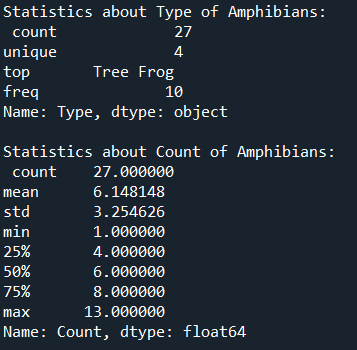top of page

# Automating Excel Data Visualizations with Python

With a basic knowledge of Python, it is possible to write code that automatically pulls data from excel sheets, analyzes the data, and provides desired visualizations in seconds. Reach out to us at info@depotanalytics.co if you need help designing systems in your business that solve your biggest problems.

To illustrate some basic analysis tools, we're going to analyze a mock excel spreadsheet with information about different amphibians collected over a two week period. The top of the excel sheet is below:Importing Python packages and connecting to your excel sheet:

```import pandas as pd
import matplotlib.pyplot as plt

file_path = r"Amphibian_Data.xlsx"

Connecting the file path to the function "pd.read_excel" allows Pandas to open the specified excel sheet and store its contents in a DataFrame (or df). DataFrames are a 2 dimensional data structure with rows and columns that allow for easy analysis.

Note: Additional input parameters are needed if there is a header and if you want to retrieve data from specific columns. By default, Pandas will read in the excel sheet starting from A1. I want to ignore the top row because it is blank, so I input the parameter "header = 1". Since the first column is blank, I only want to retrieve the data from columns B,C,D - I input the parameter "usecols = (1,2,3)".

Quick-Check Before Beginning

Before analyzing the data, it is important to make sure that the excel sheet was correctly transferred into the DataFrame.

To check that there are the correct columns in the DataFrame:

`print(df.columns)`To see the first 5 rows of the DataFrame:

`print(df.head())`Basic Analysis Functions

#### 1. To get a list of the different amphibians:

`print("The types of amphibians captured are: ", df['Type'].unique())`#### 2. To get the number of days each amphibian is counted:

`print(df['Type'].value_counts())`#### 3. To sum the total number each amphibian species measured:

```df_sum_animals = df.groupby(["Type"]).Count.sum().reset_index()
print(df_sum_animals)```Note: It is often helpful to copy the desired information a new DataFrame to allow for easier manipulation and to prevent accidentally altering the original data.

#### 4. To generate a bar chart:

```#Bar chart with total count of each amphibian
plt.bar(df_sum_animals['Type'], df_sum_animals['Count'], color=['black', 'green', 'blue', 'cyan'])

#Labeling chart axis
plt.xlabel(xlabel= "Amphibian Type")
plt.ylabel(ylabel= "Total Count")
plt.title(label = "Total Amphibian Count")```#### 5. To generate a histogram:

```#Grouping data by number of amphibians captured each day
df_sum_animals_by_day = df.groupby(["Date"]).Count.sum().reset_index()
plt.hist(df_sum_animals_by_day["Count"], edgecolor='black')

#Labeling chart axis
plt.xlabel(xlabel= "Total Count by Day")
plt.ylabel(ylabel= "Number of Occurrences")
plt.title(label = "Amphibian Count Distribution")

#Manually setting number of x indexes based on range of df column
plt.xticks(np.arange(min(df_sum_animals_by_day["Count"]),
max(df_sum_animals_by_day["Count"])+1, 2.0))```#### 6. To generate stacked bar chart:

```#Reset Date column to only contain day/month/year
df['Date'] = df['Date'].dt.strftime('%d/%m/%Y')

#Grouping by both date and type of amphibian and setting y axis to count
df.groupby(['Date','Type']).sum().unstack().plot(kind='bar',y='Count', stacked=True)

#Labeling chart axis
plt.xlabel(xlabel= "Total Count by Day")
plt.ylabel(ylabel= "Number of Occurneces")
plt.title(label = "Amphibian Count Distribution")```Note: When transferring dates from Excel to Pandas, it is common for a time to be added to each date, even if they are not in excel. An easy way to remove the times is using the Pandas "dt.strftime" function and specifying the desired format of the dates. Without the application of the function, the chart would look like:#### 7. To get basic statistics:

```stats_numeric_type = df['Type'].describe()
print ("Statistics about Type of Amphibians: ", "\n" , stats_numeric_type , "\n")

stats_numeric_count = df['Count'].describe()
print ("Statistics about Count of Amphibians: ", "\n" , stats_numeric_count)```Note: The type of information the "describe" function provides differs depending upon the type of data it is analyzing. The "Type" column contains string data and the "Count" column contains float data.

#### Get your excel data working for you!

If you need some help making your Excel data work for you, reach out to us at info@depotanalytics.co to schedule a free consultation!